A Hybrid Optimization Technique Applied to the Intermediate-Target Optimal Control Problem | OMICS International
Global Journal of Technology and Optimization

# A Hybrid Optimization Technique Applied to the Intermediate-Target Optimal Control Problem

Clay J Humphreys*, Richard G Cobb, David R Jacques and Jonah A Reeger

Air Force Institute of Technology, Wright Patterson AFB, OH, USA

*Corresponding Author:
Clay J Humphreys
Air Force Institute of Technology
Wright Patterson AFB, OH, USA
Tel: 9372556565
E-mail: [email protected]_t.edu

Received date: August 02, 2016; Accepted date: August 16, 2016; Published date: August 22, 2016

Citation: Humphreys CJ, Cobb RG, Jacques DR, Reeger JA (2016) A Hybrid Optimization Technique Applied to the Intermediate-Target Optimal Control Problem. Global J Technol Optim 7:200. doi:10.4172/2229-8711.1000200

Copyright: © 2016 Humphreys CJ, et al. This is an open-access article distributed under the terms of the Creative Commons Attribution License, which permits unrestricted use, distribution, and reproduction in any medium, provided the original author and source are credited.

Visit for more related articles at Global Journal of Technology and Optimization

#### Abstract

The DoD has introduced the concept of Manned-Unmanned Teaming, a subset of which is the loyal wingman. Optimal control techniques have been proposed as a method for rapidly solving the intermediate-target (mid-point constraint) optimal control problem. Initial results using direct orthogonal collocation and a gradient-based method for solving the resulting nonlinear program reveals a tendency to converge to or to get `stuck’ in locally optimal solutions. The literature suggested a hybrid technique in which a particle swarm optimization is used to quickly find a neighborhood of a more globally minimal solution, at which point the algorithm switches to a gradient-based nonlinear programming solver to converge on the globally optimal solution. The work herein applies the hybrid optimization technique to rapidly solve the loyal wingman optimal control problem. After establishing the background and describing the loyal wingman particle swarm optimization algorithm, the problem is solved first using the gradient-based direct orthogonal collocation method, then re-solved using a hybrid approach in which the results of the particle swarm optimization algorithm are used as the initial guess for the gradient-based direct orthogonal collocation method. Results comparing the final trajectory and convergence time, demonstrate the hybrid technique as a reliable method for producing rapid, autonomous, and feasible solutions to the loyal wingman optimal control problem.

#### Keywords

Hybrid; Particle swarm optimization; Direct orthogonal collocation; Loyal Wingman; Optimal control

#### Introduction

Manned-unmanned teaming (MUM-T) is a concept highlighted by multiple DoD documents [1-3] and includes the concept of the loyal wingman- an unmanned aerial vehicle under the tactical command of a manned lead. A definition and candidate scenario were established in  and it was proposed that optimal control techniques could be used to rapidly and autonomously determine the control for the intermediate-target optimal mission path that successfully avoids threats and/or minimizes time exposure to threats when they cannot be avoided.

Direct orthogonal collocation was chosen as an appropriate outer-loop solution technique for rapidly solving the intermediatetarget optimal control problem . This technique provides errorminimizing convergence to locally optimal solutions, but the nonlinear, non-convex nature of the loyal wingman problem may result in any number of locally optimal solutions. Although direct methods may contribute, the primary reason for the challenge is brought about with the use of a gradient-based Nonlinear Programming (NLP) solver. As mentioned by De La Mata , the NLP solver has a tendency to converge on the locally optimal solution that is in the region of the initial guess supplied to the NLP. Gradient-based numerical methods, however, are not the only numerical methods available for solving optimal control problems. Rao  broadly categorizes numerical methods for solving optimal control problems into gradient and heuristic methods. Gradient methods utilize derivative information provided in the problem formulation to search deterministically for the optimal solution and are local optimization methods, meaning the results converge to locally optimal solutions. Heuristic methods on the other hand begin with a set of possible solutions and use a stochastic search to continually update and adapt the initial set of solutions until an optimal solution is found. Contrary to the gradient-based methods which converge to locally optimal solutions, heuristic methods are a global technique which has a good opportunity to converge toward the correct global region.

Conway’s  survey suggested the best method was either heuristic algorithms alone or heuristic algorithms in combination with transcription techniques which utilize the gradient-based NLP. Englander  and Chilan  used a heuristic technique to generate the outer-loop solution. Englander continued to use a heuristic technique to generate the inner-loop solution, while Chilan used a gradient-based technique for the inner-loop solution. Showalter  and Vinko  used hybrid heuristic optimal control techniques for space-based applications. Modares  performed an experiment comparing various hybrid techniques and concluded that a modified heuristic particle swarm optimization combined with a gradient-based sequential quadratic program is robust and accurate when compared with other methods. Additional works [5,13-16] have used a hybrid PSO with gradient-based NLP.

The contribution of this work is demonstration of the application of the hybrid technique for producing rapid, autonomous and feasible solutions to the loyal wingman optimal control problem as defined in . A loyal wingman PSO algorithm is described which defines a particle and highlights methods for producing seeds deterministically, handling constraints and calculating costs, which all aid in providing a rapid, autonomous, feasible solution. Demonstration of this hybrid technique using the loyal wingman PSO algorithm with a 2D model in a static threat environment provides confidence in the methodology when applied to ongoing work for future publication. Future work includes using a 3D model, applying moving, stochastic threats, and the ability to dynamically re-plan the mission in the midst of a changing mission environment, such as a `pop-up’ threat.

The work herein begins by recalling multiple scenarios that will be solved using this hybrid approach and formulating the optimal control problem for the multiple scenarios. Section 3 provides a background on direct orthogonal collocation and demonstrates how various locally optimal solutions result from various initial guesses supplied to the NLP. Section 4 provides background on the particle swarm optimization and exploits the myriad variations of the PSO to produce a PSO algorithm tailored to the loyal wingman optimal control problem with the specific task of providing a rapid solution in the correct global region to supply as an initial guess to the NLP. Then, a simulation is run and metric established to compare the speed and accuracy of the hybrid technique to using DOC alone. Conclusions and recommendations for future work are provided in the final section of the document.

#### Optimal Control Problem Formulation and Scenarios

The candidate scenarios and an optimal control problem formulation were provided in a previous work  but are summarized here for convenience. A single unmanned aerial vehicle (the loyal wingman) under the tactical command of a manned lead must overfly an intermediate target (single waypoint) and rendezvous at a specified location. Threats in the area of responsibility must be avoided or if unavoidable due to a fortified target, time of exposure must be minimized. Four scenarios will be demonstrated through simulation:

1. Fixed time to fixed location with unavoidable threats

2. Minimize time to fixed location with unavoidable threats

3. Fixed time to fixed location with avoidable threats

4. Minimize time to fixed location with avoidable threats

The two-dimensional equations of motion serving as dynamic constraints in the optimal control problem are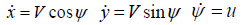(1)

Where x and y are the UAV’s x- and y- positions and ψ is heading. In order to avoid unachievable, instantaneous changes, the heading is made a state and the heading rate, rad/min is made the control, u. V is the velocity, held constant.

Boundary conditions and the intermediate target position are identified as: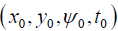- established as initial conditions (2a)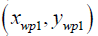- established as intermediate target condition (2b)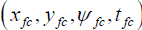- established as final conditions. (2c)

Threats, modeled using super-quadrics , are formulated as a running cost using the modified inside-outside product function in order to handle both avoidable and unavoidable threat scenarios ,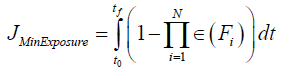(3)

∀ threats, i = 1...N, where ∈ is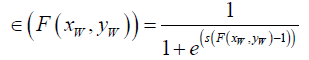(4)

where stiffness s balances optimization convergence time with threat modeling error, and F is defined as,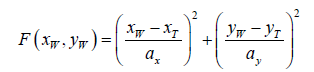(5)

where xW and yW indicate the position of the loyal wingman along its trajectory, xT and yT indicate the center point of the threat, and ax and ay are the axes lengths of the ellipse modeling the threat region. A minimizes control component is formulated to ensure a smooth control output and reduce the likelihood of control saturation: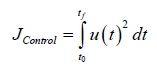(6)

By adding the minimize exposure and minimize control components and applying a convex weighting, the cost function formulation of a fixed time scenario is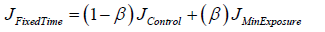(7)

Weights are chosen to ensure minimize exposure is a priority, while also keeping control smooth and maneuverable. The minimize time scenarios are formulated by adding the final condition component tf to the JFixedTime component and applying a second convex weighting, α, in Equation 8. This weighting is chosen to ensure a priority of minimizing exposure, followed by minimizing time.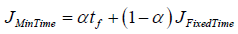(8)

#### Direct Orthogonal Collocation

Direct orthogonal collocation is a method for transcribing an optimal control problem into a nonlinear program. This method approximates the states and controls with interpolating polynomials, where in order to minimize the interpolation error between the points, the interpolation conditions are enforced at the roots or extrema of orthogonal polynomials. These approximations are substituted into the optimal control problem directly, rather than the necessary conditions for optimality, resulting in an NLP. Using commercially available solvers such as SNOPT  or IPOPT , the NLP is solved using a gradient-based search method like sequential quadratic programming (SQP) or by using an interior point method. More on the use of direct orthogonal collocation techniques for solving optimal control problems can be found in Benson  and Huntington . The work herein will use the Gauss Pseudospectral Optimization Software (GPOPS II)  which is a multi-purpose MATLAB® -based  transcription software. GPOPS II uses the roots of the Legendre polynomial as discretization points as well as choosing the Radau Pseudospectral Method (RPM) which places collocation nodes at the initial condition and in the interior, but not at the final condition. The intermediate-target optimal control problem includes an intermediate target or waypoint, which Jorris  showed is appropriately handled using multiple phases. An equality constraint is established that ensures the final conditions for phase one is set equal to the initial conditions for phase two. Additionally, state, control and time minimums and maximum limits are supplied to form the Jacobian. Nine initial guesses are used in each scenario, represented in Figure 1. A sample of the locally optimal solutions returned for the fixed time, unavoidable threats scenario is shown in Figure 2. In all four scenarios these initial guesses return multiple locally optimal solutions, a challenge the hybrid technique discussed in this paper will ameliorate.

#### Loyal Wingman Particle Swarm Optimization Algorithm

Particle Swarm Optimization was introduced by Kennedy and Eberhart in 1995 . Based on the behavior of flocks (or swarms) of birds, each particle, which is in general any scalar or vector quantity the user desires, is own through space in search of the optimal solution with respect to a defined fitness or cost function. The basic PSO algorithm is two lines of code, however in order to use those two lines of code, the loyal wingman optimal control problem must be adjusted to ensure a rapid, autonomous solution to the hybrid optimization technique. The loyal wingman PSO algorithm is described using a flowchart in Figure 3, which is broken into two major sections: Algorithm initialization and algorithm iterations.

PSO seeds and initialization

Referring to Figure 3, the first section is the production of seeds and initialization of the components of the basic two-line PSO algorithm used in the loyal wingman PSO algorithm,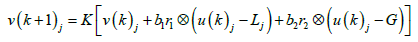(9a)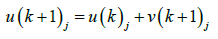(9b)

∀j =1:M seeds, ∀k =1: R iterations, where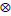represents elementwise multiplication of vector components and r1; r2 represent vectors of nondeterministic evenly distributed parameter weighting ∈[0,1] The remaining components, v, u, L, G, b1, b2, and K, are described next. There are various ways to define a seed and a recommendation of this research is to explore other methods for increased efficiency. However, the work herein defines a seed as a vector of discrete control inputs, u = [u1; u2; …uN]T, where N is the number of discrete control inputs. Simulation of the heading rate control vector, u, produces a trajectory for use in evaluating the cost and constraints. A deterministic method, described in Appendix A, was developed for producing seeds based on the following criteria: satisfy target and end point constraints as recommended by Hu and Ebert , create a broad range of possible trajectories to aid in the PSO stochastic search, and produce in a computationally efficient manner. Using this deterministic method, an initial set of M control vectors is produced u(0)1xM = [u(0)1; u(0)2; …u(0)M], which when simulated produce an initial set of M discrete vectors for each of the 3-states,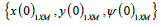.

The PSO algorithm is initialized by assigning each initially produced seed as its own current local best, Lj = u(0)j, ∀j =1:M . The cost associated with each seed, described later in this section, is determined and the index of the particle with the best cost is assigned j*, such that the global best is G = Lj*.

The first component of Equation 9 is the constriction factor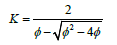(10)

With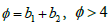suggested by Clerc  where b1 and b2 represent the local (L) and global (G) factors. The choice of these parameters effect the global and local nature of the search as well as convergence tendencies . These values were chosen for use in the loyal wingman PSO algorithm through an experiment described in Appendix B.

Finally, the velocity component v(0)j is initialized to 0, ∀j =1:M . There are many ways to initialize a PSO algorithm and additional work could be done to tune the various parameters for a given set of scenarios. This work does not claim to have found the perfect combination for optimal performance; however, the initialization described in this section is sufficient to accomplish the desired task, which is to provide a rapid initial guess to the gradient-based NLP those results in a feasible solution. Additional work in determining the right seeds and parameters may further improve the quality and efficiency of the results.

PSO iterations

After the PSO is initialized, a series of steps occur which allows the `flock’ of control vectors, or `particles’, to change values through a stochastic search of the space, moving toward the correct global region. Beginning with the first iteration, k = 1, a single particle, uj, is updated through Equation 9. The updated particle is simulated using the discrete equations of motion,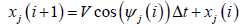(11 a)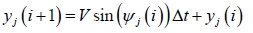(11b)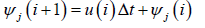(11c)

∀i =1 : N-1 where V is the velocity, held constant, and Δ t remains fixed for all particles and iterations.

The updated particle is then evaluated in Figure 3, Box 6, to check constraint criteria. The Euclidean distance from all points in the trajectory to the intermediate waypoint are calculated by,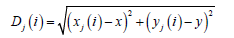(12)

∀i =1 : N . If the minimum D(i ), ∀D(i), 1: N is less than the D* threshold for meeting the intermediate waypoint constraint, then the same formulation is used to check the final condition constraint. If either constraint threshold is not met, the PSO velocity component is set to 0, vj = 0  and the next particle is evaluated ∀j =1 : M

When a particle meets both intermediate and final condition constraints, its cost is then evaluated in Figure 3, Box 7. A separate cost function is established for each scenario:

1. Minimize time to fixed location, JMinTime, (Equation 8).

2. Fixed time to fixed location, JFixedTime, (Equation 7).

The running cost is developed as the combination of minimize threat exposure and control,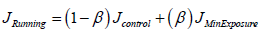(13)

where JMinExposure and Jcontrol were described in Equations 3 and 6, respectively. A minimize time to fixed final point is then just the sum of final cost and running cost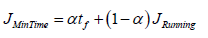(14)

A fixed time, tfc, scenario contains an additional constraint. Using a method recommended by Sedlaczek , instead of checking to ensure the constraint is met on each iteration (Box 6), the fixed time constraint is added as a component to the cost function ,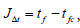such that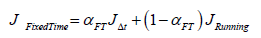(15)

The convex weighting αFT is adjusted to put increased emphasis on the fixed final time, such that, when the constraint is not met, the cost is high and if the constraint is met, this component goes to zero and only the running cost remains.

The cost of the current particle is then evaluated according to the appropriate cost scenario using Gaussian quadrature and then compared to the cost of the particle’s current local best, Lj in Figure 3, Box 8. If the updated particle’s cost is lower, then the particle’s loc al best is updated, Lj = uj in Figure 3, Box 9. The process for a single iteration, k, is repeated for all particles j = 1 : M, which is: update particle through Equation 9 (Box 4), simulate using Equation 11 (Box 5), check constraint criteria (Box 6), evaluate cost (Box 7), and update local best (Boxes 8 and 9). After all particles have been updated and evaluated (Box 10), if any local bests have been reassigned, then the costs of the new local bests are compared to the current global best, G, and if a particle’s local best cost is lower, Jbest, the global best is updated, G = Ljbest (Box 11). This completes one iteration of the PSO algorithm. The iterations continue ∀k =1-R until the iteration limit has been reached. The global best, G at the final iteration is the solution used as the initial guess to supply to the gradient-based NLP.

#### Results

The loyal wingman optimal control problem is solved using direct orthogonal collocation (DOC) and a gradient-based NLP, then re- solved using a hybrid technique in which the output from the PSO algorithm is used as an initial guess for DOC. The experiment begins by choosing the first scenario, fixed time to fixed location. Using the nine initial guesses indicated in Section 3, the DOC method was run nine times using the GPOPS II software, producing anywhere from 4 to 9 different locally optimal feasible solutions as indicated from the output from the NLP solver. The costs of these different solutions were compared and the lowest cost output was identified as the `best’ solution from the DOC method. Computation time for the DOC method is considered as the total time it takes to run GPOPS II and the NLP solver for all nine initial guesses.

Next, the loyal wingman PSO algorithm was run for a predetermined 100 iterations. The cost and computation time were captured along with a graphical representation of the global best solution when the 100 max iteration limit was achieved.

Finally, a hybrid technique is tested by taking the output of the previously mentioned loyal wingman PSO algorithm and supplying it as the initial guess into the DOC’s gradient-based NLP. The cost output is captured and the computation time is computed as the combined time to run the PSO, and the DOC with the PSO output as the initial guess. The experiment is then repeated for the remaining three scenarios. Four figures are provided which each overlay the trajectory results from the DOC method alone (dotted line), the PSO method alone (dashed line) and the hybrid method (solid line). A table in each figure identifies the cost and computation time associated with each method.

In general, it is expected that when threat regions are avoidable, each method should find trajectories that successfully avoids the threat regions. In cases were threat regions are unavoidable, it should be expected that the best way to minimize time of exposure for a constant velocity vehicle and equally weighted threat regions is to traverse the threat regions by way of a perpendicular bisector of the threat intersection points. Minimum-time scenarios should result in trajectories which are direct, while with fixed-time scenarios it is expected that additional turns in the trajectory allow for idle time in order to meet the fixed-time constraint.

The DOC method uses 40 nodes in each of two phases, for a total of 80 nodes. Figure 4 provides a heading and control rate plot that is representative of all four scenarios. The desire for smooth and continuous control maneuverability is achieved primarily through a heavily weighted minimize control component of the cost function, Equation 7. These control outputs may be varied by changing the weighting from Equation 7, and would then be subsequently bound by the established control bounds.

Figure 5 represents scenario one in which the vehicle must overfly an intermediate target in a layout in which threats are not avoidable, and conclude with rendezvous at the fixed time and specified location. All three methods find trajectories that minimize exposure through unavoidable threat regions with a perpendicular bisector and the DOC method produces a trajectory with a lower cost than does the PSO alone. However, the hybrid method produces the lowest cost solution at a computation time that is less than the DOC method alone.

Figure 6 represents the results of scenario two in which the vehicle must overfly an intermediate target in a layout which threat regions are unavoidable and conclude at final rendezvous in minimum time. The DOC solution produces a lower cost solution than the PSO method but takes nearly twice the computation time. The hybrid method produces the exact same solution as the DOC method alone, but does so in a much more computationally efficient manner. The time it takes to run the hybrid method is nearly 30% faster than the DOC method alone.

Figure 7 represents the results of scenario three in which the vehicle must overfly an intermediate target prior to a fixed final time and location rendezvous. This mission can be accomplished without exposure to any threat regions and all methods do so successfully. The results are similar to the scenario previously discussed where the cost of the DOC solution is lower than the cost of the PSO solution, but to run the DOC solution nine times, once again takes twice the computation time. In this case, the hybrid method outputs the same trajectory and cost associated with the DOC method alone, but does so 12 seconds faster, in approximately 40% of the time it takes to run the DOC method alone. Figure 8 is scenario four, representing a vehicle that must traverse an intermediate target prior to rendezvous at a final location. The mission may be accomplished without exposure to threats, which all methods obtain successfully. What is interesting about this scenario is the DOC method by itself returns a trajectory that lies North (positive y-direction) of the isolated threat on its way to the final rendezvous. This trajectory adds unnecessary cost in a minimize time problem and its result is a good example of one of the challenges faced by the gradient-based search methods. As the NLP takes small steps in a direction to minimize the time, the trajectory becomes exposed to the threat region and bounces back above, effectively being caught in a local minimum. The global and stochastic nature of the PSO results in a more direct, time- saving route but did not converge once it found the global region. When this PSO result is supplied as the initial guess into the NLP in a hybrid approach, a lower-cost solution is found in less than half the computation time to run the DOC method alone. Table 1 provides a comparison of cost and computation time (in seconds) for all three methods for each of the four scenarios.

Scen 1 Scen 2 Scen 3 Scen 4
Cost Time Cost Time Cost Time Cost Time
PSO 17.588 14.981 2.806 17.680 2.797 14.323 1.560 17.745
DOC 11.194 25.242 2.251 29.159 2.139 28.159 1.539 52.948
Hybrid 9.243 17.410 2.251 20.193 2.139 16.867 1.421 20.320

Table 1: Cost and computation time (in seconds) for all scenarios.

#### Conclusions

This work demonstrated application of the hybrid technique to provide rapid, autonomous, and feasible solutions to the loyal wingman optimal control problem. Direct orthogonal collocation is a rapid method for solving optimal control problems, but a challenge is picking an initial guess for the gradient-based NLP that converges rapidly without getting `stuck’ in an undesirable locally optimal region. Heuristic methods present the opposite challenge; they are relatively fast at finding the global region, but may not converge to the globally optimal solution. A hybrid technique recommended by Conway  and used by others is a way to rapidly develop an initial guess in the global region, which the NLP can then rapidly solve to find the best locally optimal solution. The loyal wingman PSO algorithm was developed for use in the loyal wingman application, to include producing seeds deterministically in order to decrease PSO computation time and applying methods of handling constraints recommended by other authors.

Results indicated the DOC solution was dependent upon the initial guess as was suggested, producing multiple locally optimal solutions. If a good initial guess was not supplied, then it is questionable whether the NLP would converge on a desirable solution. One run of the DOC method is fast, but if the user doesn’t have a good initial guess and therefore needs to run the method multiple times, then the computation time is high. The PSO algorithm used for this application was fast and found solutions in a desirable region. When the PSO solution was supplied to the NLP in a hybrid method, the result was consistently even with or better than the DOC method alone. In all cases, the hybrid method produced results faster than the DOC method alone.

Although challenging threat scenarios were established, it is possible the deterministic seed production and the choice of parameters are tuned to the specific scenarios of this work. A recommendation for future work is to continue adjusting the loyal wingman PSO to increase the stochastic search region, ensuring randomly generated threat scenarios are solvable, as well as improving the computation time. A second recommendation for future work is to study the definition of a particle in this application as the coefficients to a polynomial, discretized at the roots of an orthogonal basis set. There is potential for synergy by using the DOC transcription of the optimal control problem and applying it for use in the PSO. A third recommendation is to change the scenario to require rendezvous with additional intermediate waypoints applied with random distribution.

While attempting to rapidly and autonomously solve the intermediate target optimal control problem using direct methods, supplying a good initial guess has consistently been a challenge. The hybrid technique was shown in this work to ameliorate that challenge and these results show a promising method for solving future planned work which includes a 3D dynamic model, moving stochastic threats, and dynamic re-planning in the midst of a changing mission environment.

#### Appendix A: Deterministic Method to Produce PSO Seeds

For an optimal control problem, a particle of a PSO algorithm is a vector of discrete time control inputs that produce an optimal mission path with respect to the identified cost function. The loyal wingman PSO was seeded using a deterministic algorithm with a goal of meeting the following criteria: meet target and endpoint constraints, represent a broad range of possible trajectories to aid the PSO’s stochastic search, and achievable in a computationally efficient manner.

The first step is to produce a set of possible two-dimensional trajectories with Euclidean state space (x,y) data alone, using a spline interpolation. Initial, intermediate target, and final conditions, denoted p1, p2, and p3, are criteria established in the problem scenarios and provide a means to ensure the spline fit data meets constraint criteria. For purposes of this discussion, consider phase 1 between p1 and p2, and phase 2 as between p2 and p3. In order to allow for a broad range of trajectories, intermediate points are chosen in each of the phases. The Euclidean distance connecting p1 to p2 and p2 and p3 is computed as d1 and d2, respectively. Beginning with phase 1, a perpendicular bisector L1, the length of d1 is constructed at the midpoint between p1 and p2. Points are now chosen on this perpendicular bisector to add curvature to the splines. If for example, α points are chosen on L1, then there are α curves which can now connect p1 to p2. Using the same method, sample points are also chosen in phase 2, such that if β points are chosen there are β curves which can now connect p2 to p3. When the two phases are combined there are now M = α * β possible splines that can be constructed that meet initial, intermediate target, and endpoint location criteria. The constructed points can be seen visually in Figure 9.

After the points through which a spline interpolation is desired have been identified, the two-dimensional (x,y) points are parameterized using Eugene Lee’s centripetal scheme,  through a convenient MATLAB function cscvn, which is the accumulated square root of the chord length. The MATLAB function spline can then be used which allows for specification of derivative conditions at the boundaries. In the case of the loyal wingman, the derivative boundary condition is heading, ψ , computed as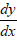. Any initial heading can be supplied in the construction of the spline by specifying the initial condition boundary as y = sin (ψ ) , x = cos (ψ ) . Specification of the boundary condition is very important in the loyal wingman PSO because the algorithm will simulate the controls with an identified initial heading. If the spline fit does not return an initialequivalent to the initial condition specified by the loyal wingman problem, then the simulation will not produce a trajectory that meets constraints. Choosing α = β = 7 and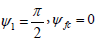the returned 49 spline fit curves can be seen in Figure 10. No consideration is given to avoiding threats when producing these trajectories. This allows for the deterministic production of seeds as a general algorithm that can be used as the loyal wingman optimal control problem scenarios are varied.

At this point, a specified set of (x,y) points (in this case 100 evenly spaced points for each spline) have been identified through a parameterized spline interpolation, but the goal is to achieve a set of controls as particles in the PSO algorithm. This is achieved by using the data output from the spline function to derive heading and heading rate. Before this can be done, the (x,y) data must be re-parameterized to time. The arc length between each (x,y) data point is computed using Equation 16,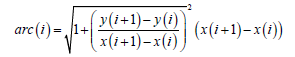(16)

Then divided by the loyal wingman’s constant velocity to achieve a time parameterization of the (x,y) data points. The (x,y) data points are then re-discretized using a spline interpolation to evenly spaced time units. There are now 49 trajectories composed of evenly spaced (x,y) data points parameterized to time. From this, heading can be derived at each time step i using,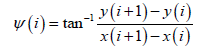And finally heading rate can be derived at each time step through the discrete equation of motion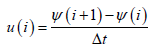The desired particles, heading rate control vectors, have now been achieved. However, the time step for each trajectory is slightly different and in order to fit into the loyal wingman’s PSO architecture, the time steps must be equivalent for all trajectories. A common vector length, chosen based on the longest trajectory and even time step is chosen to which all control vectors are re-fit. In cases where the common vector length is beyond what is needed to simulate the trajectory, the remaining elements are filled with 0. This allows the length of the trajectory to grow and shrink to the tune of the PSO algorithm’s iterations.

#### Appendix B: Experiment to Choose PSO Parameters

The PSO algorithm contains various parameters such as social weighting and a constriction factor. Section 4 highlighted convergence tendencies associated with the choice of parameters. An experiment was performed to determine the most appropriate value for the constriction factor as well as the choice of b1 and b2 for use in the loyal wingman optimal control problem.

The value φ was varied from 4.1 to 7 along with the associated constriction factor as determined from Equation 10. The PSO algorithm was run for each value of φ 10 times and the average cost and time to converge were recorded. The results can be seen in Table 2. The best cost in this experiment was with a φ of 4.2 and constriction factor of 0.6417. φ is a sum of the two social weighting factors so a separate experiment was run, where φ and K are fixed at 4.2 and 0.6417, respectively, but the individual social components, b1 and b2, are varied. Each scenario is run 10 times and the average cost is calculated and captured in Table 3. The best cost was found when b1 was at 1 or less. This result is because a high local weighting causes the search to stay in the local area, never moving its search toward the global best. When global best, b2 is weighted high, the particles search outside their local area in a movement toward the globally best particle.

K Cost Its
7.0 0.2087 2.4670 102
6.2 0.2534 2.4587 124
5.8 0.2845 2.4371 140
5.0 0.3820 2.4130 180
4.2 0.6417 2.3194 452
4.1 0.7298 2.3402 454

Table 2: Average cost of various constriction factors.

b1 b2 Cost
4.0 0.2 2.4114
3.5 0.7 2.3468
3.0 1.2 2.3420
2.5 1.7 2.3127
1.5 2.7 2.2817
1.0 3.2 2.2688
0.2 4.0 2.2751
0.1 4.1 2.2715

Table 3: Average cost of various social weighting factors.

#### Acknowledgments

The authors would like to thank the Air Force Research Laboratory, Power and Controls Division for their funding and support of this project.

#### References

Select your language of interest to view the total content in your interested language

### Article Usage

• Total views: 9936
• [From(publication date):
August-2016 - Dec 14, 2019]
• Breakdown by view type
• HTML page views : 9736Can't read the image? click here to refresh